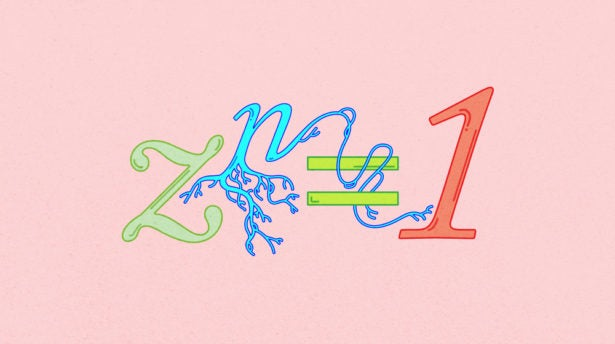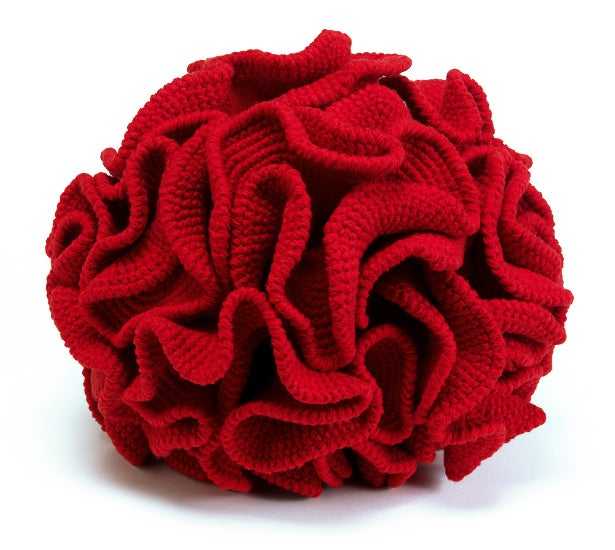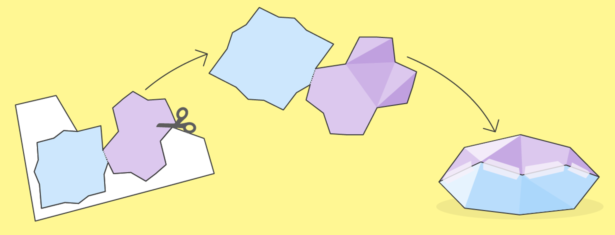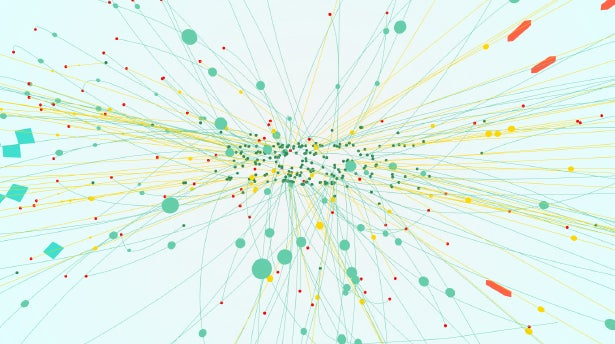# Kevin Hartnett

•Explore## The Almost-Proof of Fermat’s Last Theorem

19th-century mathematicians thought the “roots of unity” were the key to solving Fermat’s Last Theorem. Then they discovered a fatal flaw.

•Explore## The Hidden Twist to Making a Möbius Strip

The simple Möbius strip illustrates a deep mathematical challenge that has long tormented the field of symplectic geometry.

•Explore## How Curvature Makes a Shape a Shape

The ancient study of an object’s curvature is guiding mathematicians toward a new understanding of simple equations.

•Explore## Test Your Mathematical Sculpting Skills

Can you turn a two-dimensional fractal into a 3-D object? Break out your scissors and tape for a chance to win a 3-D printed sculpture.

•Explore## The Math That’s Too Difficult for Physics

How do physicists reconstruct what really happened in a particle collision? Through calculations that are so challenging that, in some cases, they simply can’t be done. Yet.